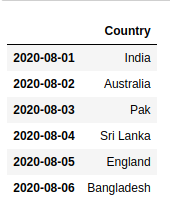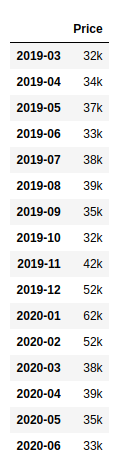# How to determine Period Range with Frequency in Pandas?

• Last Updated : 25 May, 2021

In pandas, we can determine Period Range with Frequency with the help of period_range(). pandas.period_range() is one of the general functions in Pandas which is used to return a fixed frequency PeriodIndex, with day (calendar) as the default frequency.

Syntax: pandas.to_numeric(arg, errors=’raise’, downcast=None)

Parameters:
start : Left bound for generating periods
end : Right bound for generating periods
periods : Number of periods to generate
freq : Frequency alias
name : Name of the resulting PeriodIndex

Returns: PeriodIndex

Example 1:

## Python3

 `import` `pandas as pd`     `# initialize country` `country ``=` `[``"India"``, ``"Australia"``, ``"Pak"``, ``"Sri Lanka"``, ` `           ``"England"``, ``"Bangladesh"``]`   `# perform period_range() function` `match_date ``=` `pd.period_range(``'8/1/2020'``, ``'8/6/2020'``, freq``=``'D'``)`   `# generates dataframes` `df ``=` `pd.DataFrame(country, index``=``match_date, columns``=``[``'Country'``])`   `df`

Output:Example

## Python3

 `import` `pandas as pd`   `# initialize country` `Course ``=` `[``"DSA"``, ``"OOPS"``, ``"DBMS"``, ``"Computer Network"``, ` `          ``"System design"``, ]`   `# perform period_range() function` `webinar_month ``=` `pd.period_range(``'8/1/2020'``, ``'12/1/2020'``, freq``=``'M'``)`   `# generates dataframes` `df ``=` `pd.DataFrame(Course, index``=``webinar_month, columns``=``[``'Course'``])`   `df`

Output:Example 3:

## Python3

 `import` `pandas as pd`     `# initialize gold price` `gold_price ``=` `[``"32k"``, ``"34k"``, ``"37k"``, ``"33k"``, ``"38k"``, ``"39k"``, ``"35k"``,` `              ``"32k"``, ``"42k"``, ``"52k"``, ``"62k"``, ``"52k"``, ``"38k"``, ``"39k"``,` `              ``"35k"``, ``"33k"``]`   `# perform period_range() function` `price_month ``=` `pd.period_range(start``=``pd.Period(``'2019Q1'``, freq``=``'Q'``),` `                              ``end``=``pd.Period(``'2020Q2'``, freq``=``'Q'``),` `                              ``freq``=``'M'``)`   `# generates dataframes` `df ``=` `pd.DataFrame(gold_price, index``=``price_month, columns``=``[``'Price'``])`   `df`

Output:My Personal Notes arrow_drop_up
Recommended Articles
Page :